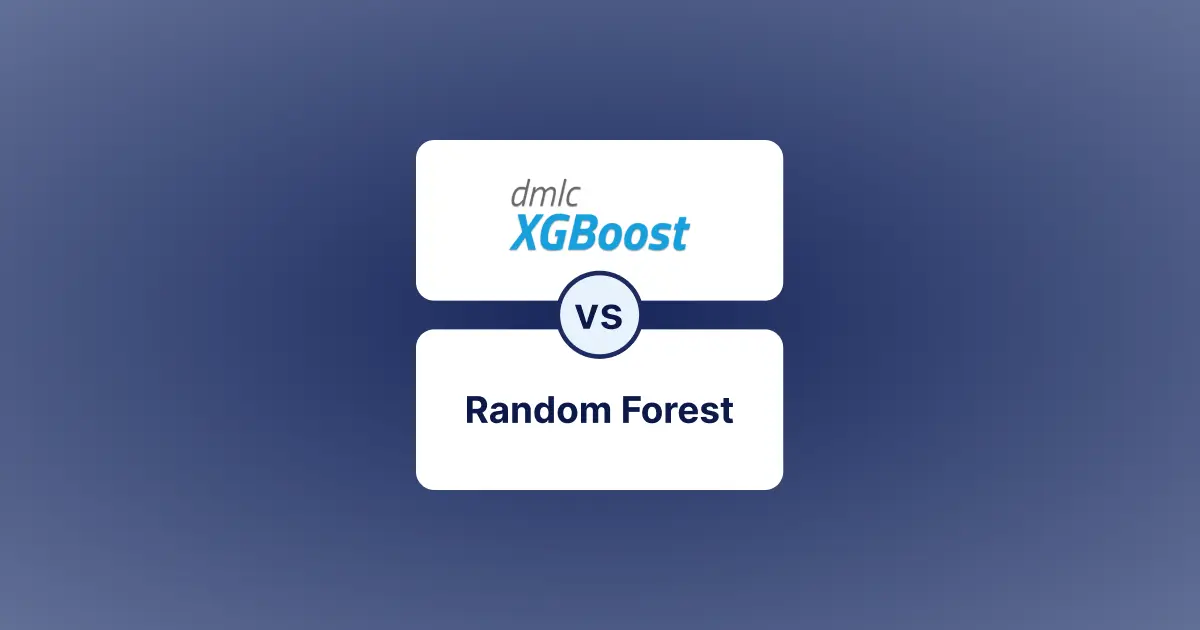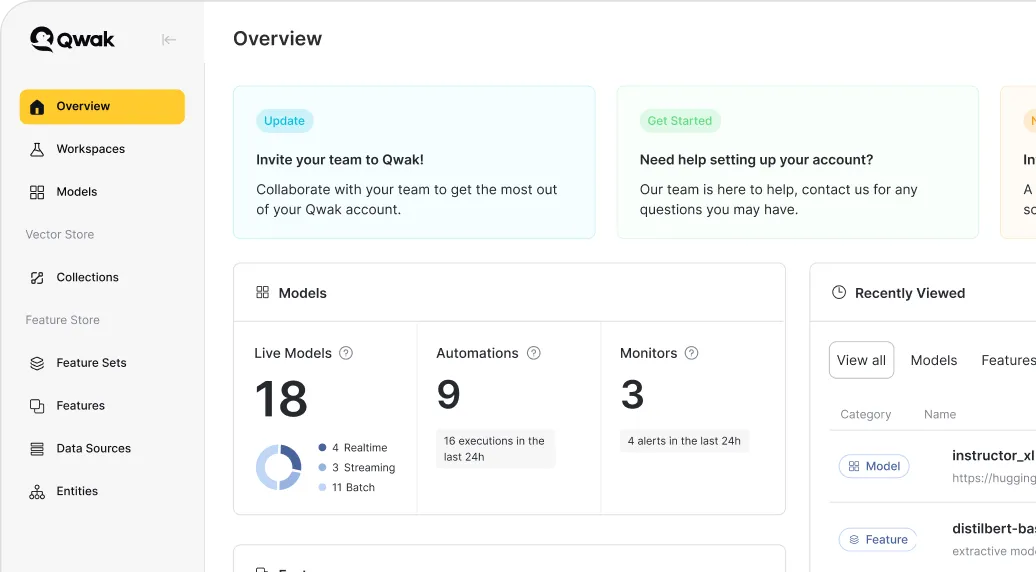# XGBoost versus Random Forest

Tree-based algorithms are highly efficient for regression and classification tasks. Let’s compare XGBoost vs Random Forest to see what benefits each offers.Alon Lev
Co-Founder & CEO at Qwak
December 19, 2022## XGBoost vs Random Forest: How to Choose?

Machine learning (ML) uses advanced mathematical techniques to find complex hidden patterns within data. These patterns offer insights that help build predictive models and help solve real-world problems. During the learning stage, the ML model explores the data repetitively, enhancing its learning with every iteration. However, even the most advanced models carry a margin of error. In this article, we will discuss XGBoost vs Random Forest and compare their architectures, features, and performance.

Machine Learning has seen significant leaps in model performance and complexity. The most basic ML algorithm is Linear Regression, which explores a linear trend within the data. Moving on from linear models, we have tree-based models, bagging models, boosting models, Support Vector Machines (SVM), and Naive Bayes. Each of these algorithms displays ground-breaking results for modern problems.

XGBoost and Random Forest are two such complex models frequently used in the data science domain. Both are tree-based models and display excellent performance in capturing complicated patterns within data. Random Forest is a bagging model that trains multiple trees in parallel, and the final output is whatever the majority of trees decide. The XG boosting algorithm creates a sequential ensemble of tree models, all of which work to improve each other and determine the final output. This algorithm can be used to create the XGBoost classifier as well as the regressor. Before moving on, let's discuss more tree and boosting algorithms.

## Tree-Based Machine Learning Algorithms

Tree-based ML models are very popular among data scientists. Tree-based algorithms form a class of supervised machine-learning models that provide high accuracy and interpretability. They develop a series of if-else statements that analyze the input features and point to the ground truths. Once created, these statements can determine outputs for unseen data.

Two of the most popular tree algorithms are the Decision Tree and Random Forest. Both of these can solve classification and regression problems. Let's understand their work in detail.

### Decision Trees

Decision Trees (DTs) analyze the input features and filter the dataset based on feature values such that the remaining data points are the ones that provide the maximum information about the target variable. Simply put, it finds the essential feature values that demonstrate maximum correlation to the output.

Say we have a dataset to determine whether the climate is hot. One of the features,' temperature,’ suggests that the output is hot whenever its value exceeds 40. This particular feature value provides maximum information gain to the ML model. The ‘Information gain’ from a feature is determined by its Gini Index Impurity. The decision tree algorithm calculates Gini Index values for all features and places them in the Tree accordingly. The lowest value feature forms the first node of the tree, and the Gini Impurity increases as we go down.

### Random Forest

The Random Forest algorithm offers an improvement over the Decision Tree. A significant problem with decision trees is overfitting the training data making them susceptible to poor performance in unseen scenarios. Random Forest solves this problem by training a collection of decision trees on a subset of the data.

The Random Forest algorithm begins by sampling the dataset on both rows and columns to create random subsets of the original dataset. The filtration procedure ensures that each feature from the original dataset makes it to at least one of the filtered datasets. Individual decision trees are trained for each dataset, and their outputs are collected. The output class obtained from most trees becomes the final prediction.

A cluster of multiple decision trees forms a random forest. Each of these trees learns from a subset of the original features. The intuition behind it is that every individual tree is a weak learner (due to exposure to partial data), and the complete forest can present accurate results while tackling high variance. This ensemble model technique is also known as Bagging.

Another common ensemble technique is Boosting. Let’s talk more

## Boosting Algorithms

While Bagging algorithms train decision trees in parallel, Boosting algorithms take a serial approach. Each model in the series trains upon its predecessor's mistakes, trying to correct them. The final model is a collection of weak learners trained on the residue of strong learners to form the final prediction.

The most basic of boosting algorithms is Adaptive Boosting (AdaBoost). It utilizes decision stumps as base learners to create an ensemble. The decision stumps classify the output variable using only one feature from the dataset. We calculate the Gini impurity index for each feature. The feature with the lowest impurity will form our first base learner (decision stump).'

Each example point for the first base learner has equal weightage while classifying the results. All misclassified examples from the first stump are passed to the next learner with a higher weightage. This continues till the last decision stump is reached. This way, each stump is trained to tackle the examples confusing the previous learner, and the result is a sequence of models forming a high-performing classifier.

Gradient Boosting (GB) works similarly to AdaBoost but with slight changes to the algorithm. Firstly, the classifiers (learners) used in GB are fully trained decision trees as opposed to decision stumps in AdaBoost. Secondly, while AdaBoost varies the weightage of each feature, GB looks to optimize the loss as the inputs travel down the sequence of learners.

The GB algorithm starts with calculating predictions using the first learner. Next, it calculates the error for the predictions using a loss function. From here on, every following learner trains to predict the loss of their predecessor. The final prediction is calculated by multiplying the predictions of each learner with a learning rate (a number usually between 0 and 1) and then subtracting the final values.

The intuition behind GB is that by subtracting the errors predicted by each tree from the actual prediction from the first learner, we can bring the value closer to the ground truth.

Extreme Gradient Boosting (XGBoost) is a C++ library that follows the same algorithm as GB and has APIs available for several languages. It is only a different implementation of the original algorithm that provides certain benefits, which we will discuss later.

The XG Boosting algorithm uses advanced regularization techniques to suppress weights, prevent overfitting, and enhance its performance in real-world scenarios. On top of this, the implementation allows the algorithm to cache data and utilize multiple CPU cores for speedy processing. The enhanced performance and speed have made XGBoost one of the most popular machine-learning algorithms in recent years.

The XG boosting algorithm can be used for classification and regression. The XGBoost classifier is used for discrete outputs (classes), while the regressor predicts continuous values.

The comparison between the XGBoost classifier and Random Forest (RF) is more like a Bagging VS Boosting debate. Although both these methods use tree-based learners, their architecture and algorithms are fundamentally different, which results in differences in performance and accuracy.

### Architecture

Random Forest, being a bagging model, generates decision trees and calculates their predictions in parallel. XGBoosting algorithm is a sequential model, which means that each subsequent tree is dependent on the outcome of the last. This architecture does not allow it to parallelize the overall ensemble. Although it may be noted XGBoost does utilize parallelization. Instead of building trees, it parallelizes node building within each tree, making the tree-building process exponentially fast.

### Performance

Both the models in question vary in performance for different use cases. Let’s analyze these below.

#### Speed

Both algorithms are efficient in their training due to the ability to utilize multiple CPU cores for parallel operations. The training speed largely depends on the hyper-parameters, i.e., the number of learners, tree depth, etc., and the training data size. This discussion ends in a draw as neither algorithm offers any significant upgrade over the other in terms of speed.

#### Accuracy and Overfitting

Upon its introduction, the RF algorithm quickly became popular due to its high accuracy. However, it wasn’t long before people realised that the high accuracy came at the cost of over-fitting, causing the model to display poor performance in real-world scenarios. RFs tend to overfit when the sampled data presents similar data points to each tree. Developers can counter overfitting by pre-processing and cleaning the data, but the challenge remains for real-time use cases.

The XGBoost algorithm does not construct trees to their full depth. It prunes trees at a certain point depending on the node's similarity score. It considers the “Gain” of a node as the difference between the similarity score of the node and its children and stops building the tree if the score is considered minimal. The smaller trees counter the overfitting problem and allow the model to generalize better. Countering overfitting means XGBoost models show better accuracies on test data making them better suited for real problems.

#### Hyperparameter Tuning

XGBoost is a significantly complex algorithm compared to RF and involves several parameters that can alter the performance. This discourages engineers from getting into the complexity of tuning the algorithm for better performance.

With that being said, the hyperparameters for XGBoost are set only for the first tree. The rest of the trees adjust themselves with every iteration using the loss of the preceding tree and carrying out gradient descent. This is beneficial in cases where the input data is real-time and displays high variation. A model whose parameters adjust itself iteratively (XGBoost) will learn better from streaming data than one with a fixed set of parameters for the entire ensemble (Random Forest).

Working With Unbalanced Data

The XGBoost model performs better than RF when we have a class imbalance. The boosting algorithm iteratively learns from the mistakes of the previous tree. So if a tree fails to predict a class (most likely the imbalanced class), the proceeding tree will give more weightage to this sample. Intuitively, this attempts to equalize the model by giving more weightage to less represented categories. The RF algorithm does not have any such mechanism to handle data imbalance.

## Productionizing Machine Learning With Qwak

Random Forest and Extreme Gradient Boosting are high-performing machine-learning algorithms, and each carries certain pros and cons. RF is a bagging technique that trains multiple decision trees in parallel and determines the final output via a majority vote. XGBoost is a boosting technique that sequentially creates decision trees, each tree improving upon the mistakes of the previous one. The final result is a sum of outputs from all the trees. XGBoost is much more complicated compared to RF. This makes it challenging to work with but adds key benefits, such as better performance, countering overfitting, and internally handling data imbalance. Due to its superior features and better real-world performance, engineers prefer XGBoost over RF.

Qwak simplifies the productionization of machine learning models at scale. Qwak’s Feature Store and ML Platform empower data science and ML engineering teams to Build, Train and Deploy ML models to production continuously.

By abstracting the complexities of model deployment, integration, and optimization, Qwak brings agility and high velocity to all ML initiatives designed to transform business, innovate, and create a competitive advantage.

Chat with us to see the platform live and discover how we can help simplify your ML journey.